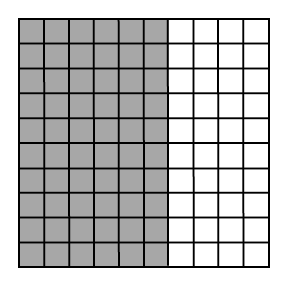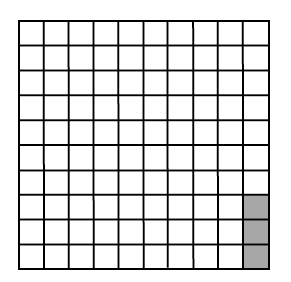### Home > CC1MN > Chapter 5 > Lesson 5.1.3 > Problem5-40

5-40.

Use the hundredths grids at right to answer the following questions.

1. Give three names for the larger shaded area.

Find the number of shaded squares by finding the area represented or by counting each square. If this number is out of $100$ total squares, how can you represent this value as a fraction, decimal, or percentage?

2. Give three names for the smaller shaded area.

See part (a).

$0.03 = 3\%= \frac{3}{100}$

3. What are two other names for $120\%$? Can you show $120\%$ on a single hundredths grid? Explain your thinking.

• How can $120%$ be represented as a fraction or decimal value?

If there are only $100$ squares on a single grid, can you represent $120$ squares on this grid?